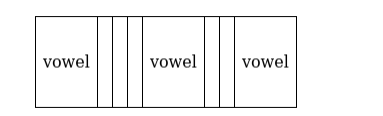# Find the number of arrangements of the letters of the word ‘ALGEBRA’

Question:

Find the number of arrangements of the letters of the word ‘ALGEBRA’ without altering the relative positions of the vowels and the consonants.

Solution:

To find: number of arrangements without changing the relative position

The following table shows where the vowels and consonants can be placed

Consonants can be placed in the blank placesThere are 3 spaces for vowels

There are 3 vowels out of which 2 are alike

Vowels can be placed in $\frac{3 !}{2 !}=3$ ways

There are 4 consonants, and they can be placed in $4 !=24$ ways

$\Rightarrow$ Total number of arrangements $=24 \times 3=72$ ways